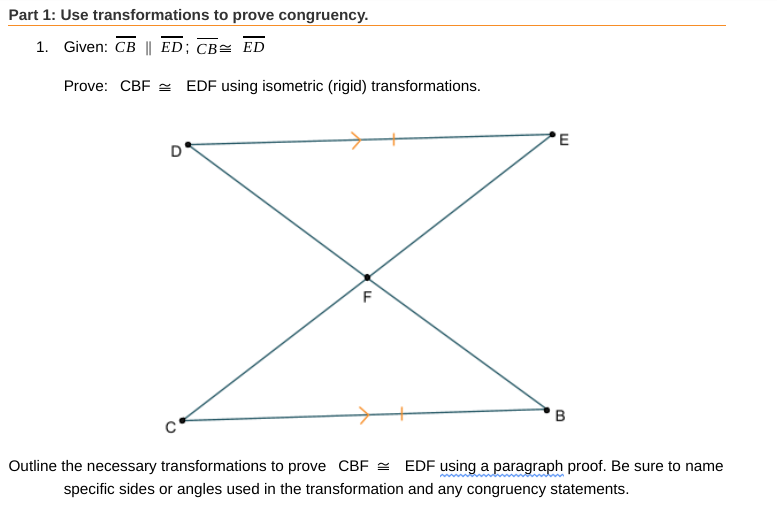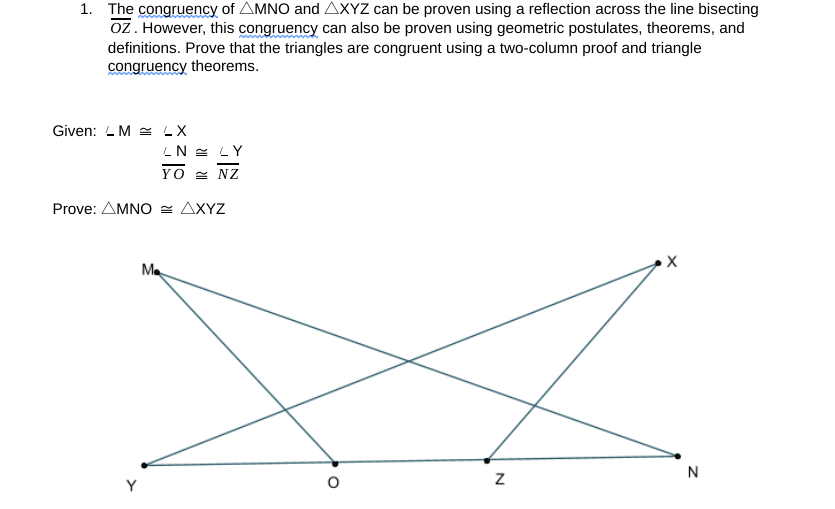# Part 1: Use transformations to prove congruency.1. Given: CB || ED; CB= EDProve: CBF = EDF using isometric (rigid) transformations.EDF using a paragraph proof. Be sure to nameOutline the necessary transformations to prove CBF =specific sides or angles used in the transformation and any congruency statements. 1. The congruency of AMNO and AXYZ can be proven using a reflection across the line bisectingoz. However, this congruency can also be proven using geometric postulates, theorems, anddefinitions. Prove that the triangles are congruent using a two-column proof and trianglecongruency theorems.Given: L M = LXLN = LYYO = NZProve: AMNO = AXYZхMe

Question
6 viewshelp_outlineImage TranscriptionclosePart 1: Use transformations to prove congruency. 1. Given: CB || ED; CB= ED Prove: CBF = EDF using isometric (rigid) transformations. EDF using a paragraph proof. Be sure to name Outline the necessary transformations to prove CBF = specific sides or angles used in the transformation and any congruency statements. fullscreenhelp_outlineImage Transcriptionclose1. The congruency of AMNO and AXYZ can be proven using a reflection across the line bisecting oz. However, this congruency can also be proven using geometric postulates, theorems, and definitions. Prove that the triangles are congruent using a two-column proof and triangle congruency theorems. Given: L M = LX LN = LY YO = NZ Prove: AMNO = AXYZ х Me fullscreen
check_circle

Step 1

As per norm, we are answering only th...

### Want to see the full answer?

See Solution

#### Want to see this answer and more?

Solutions are written by subject experts who are available 24/7. Questions are typically answered within 1 hour.*

See Solution
*Response times may vary by subject and question.
Tagged in

### Coordinate Geometry